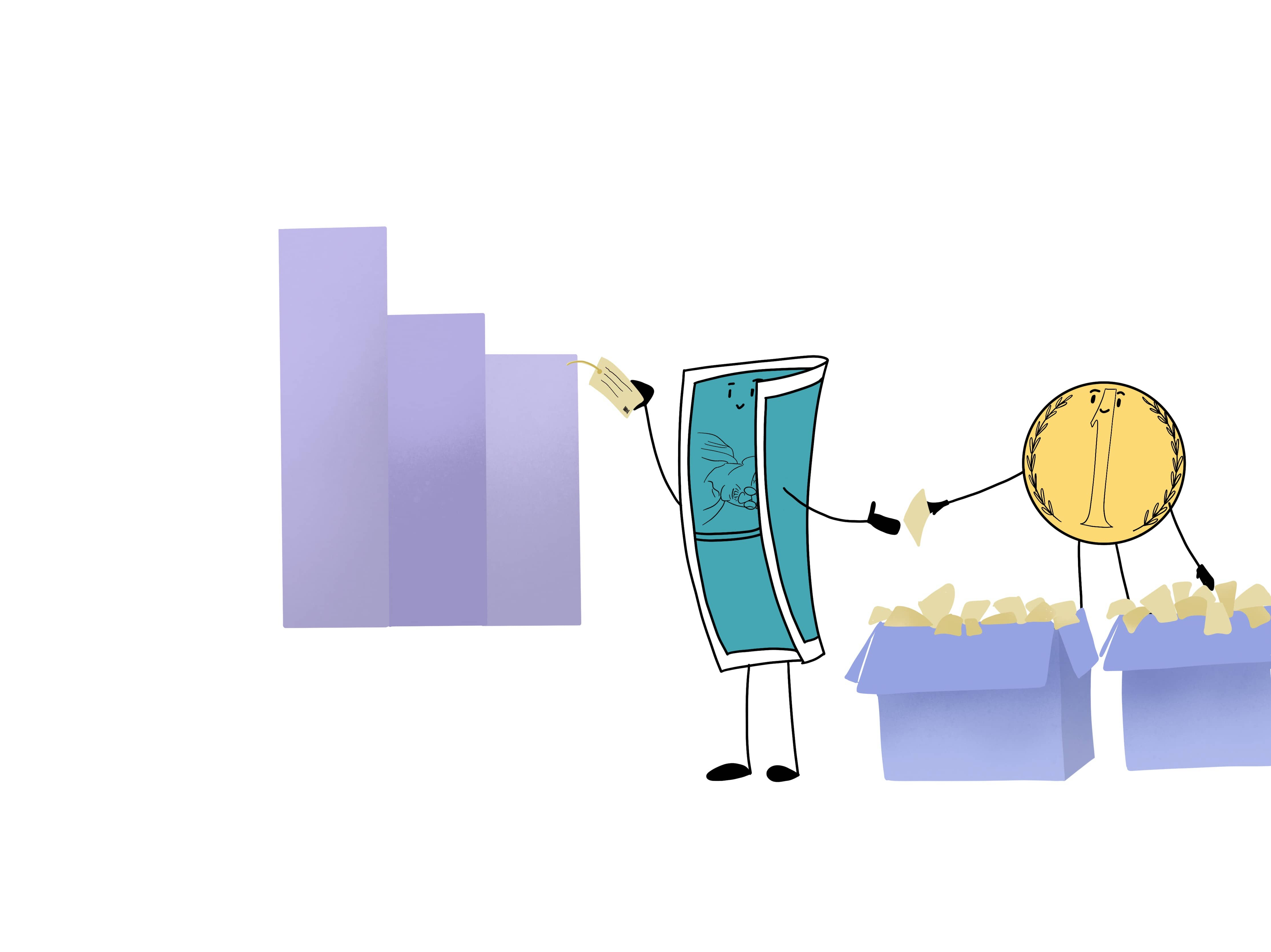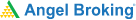# What is the Pricing Structure of Futures ContractIn the last chapters, we have learnt about margin trading, calculation of margins and the risks involved in margin trading. Let us now discuss another important topic which is the future's contract.

Let us begin by understanding what futures are. The derivative products whose value depends mainly on the price of the underlying stocks or indices are called futures. The cost of underlying stocks or indices is not direct. There is a difference between the underlying asset’s price,  cash segment, and the derivatives segment. We can understand this difference through two pricing models for future contacts. Through these, you can estimate the price of a stock future or how the index future contract might behave. The pricing models are:

• The Cost of Carry Model
• The Expectancy Model

You must understand that these models just provide a platform on which you can base your understanding of the futures prices. You need to understand these models so that you have an idea about what you should be expecting from the future price of a stock or of an index.Let us begin by understanding these models in depth.

## The Cost of Carry Model

In the Cost of Carry Model, there is no difference between the cash and the futures prices. Why is it so? It is because the Cost of Carry Model assumes that markets are perfectly efficient and removes the opportunity for traders to take advantage of price differences in two or more markets which are also called arbitrage.

In a scenario where there is no chance of arbitrage, unless an investor trades in underlying assets, he won’t know the spot and futures market price. The reason behind this is that the final earnings are the same. Therefore, we can conclude that:

Price of a futures contract (FP) = Spot Price (SP) + (Carry Cost – Carry Return)

Carry Cost means the cost of holding the asset till the maturity of the futures contract. It may include the cost of storage cost, interest paid to acquire and hold the asset, and financing costs.

Carry Return means any income which is derived from the asset while holding it in the form of dividends and bonuses etc. When we calculate the futures price of an index, the Carry Return means the average returns which are given by the index at the time of its holding period in the cash market. The net cost of carry is the net value of carry cost and carry return.

As per this model, there can be benefits of cost if the position is kept open in the cash market.Learning & Earning is now super simple₹ 0 Equity Delivery

No Hidden Charges₹ 20 Per Order For Intraday

FAQ,Currencies & CommoditiesZERO Brokerage*

on ALL SegmentsFREE Margin

Trade Funding

### Expectancy Model Of Futures Pricing

According to the Expectancy Model of futures pricing the futures price of an asset is technically the spot price of the asset which is expected to be in the future.

Therefore, the futures price of the asset will be positive if the overall market sentiment leans towards a higher price for an asset in the future.

Similarly when there is a rise in bearish sentiments in the market, then it would lead to a fall in the asset’s futures price.

Expectancy Model Of Futures Pricing model is based on the notion that there is no relationship between the asset’s present spot price and its futures price. What is significant here is what the expected future spot price of the asset is going to be.

This is the reason why a lot of stock market participants look towards the trends in futures prices to anticipate the fluctuation in price fluctuation in the cash segment.#### Wrapping up

Now that you understand how to price a future’s contract, it’s only logical that we move on to the next big topic - Nitty Gritties of margin calculations. To discover the answer, head to the next chapter.

#### A Quick Recap

• The derivative products whose value depends largely on the price of the underlying stocks or indices are called futures.
• There are two pricing models which are used to estimate the price of a stock future or how the index future contract might behave- Cost of Carry Model and Expectancy Model.
• The Cost of Carry Model assumes that markets are perfectly efficient and removes the opportunity for traders to take advantage of price differences in two or more markets which is also called arbitrage.
• Carry Cost is the cost of holding the asset till the maturity of the futures contract.
• Carry Return means any income which is derived from the asset while holding it in the form of dividends and bonuses etc.
• As per the Expectancy Model of futures pricing the futures price of an asset is technically the spot price of the asset which is expected to be in the future.Test Your Knowledge

Take the quiz for this chapter & mark it complete.

Comments (0)

Add Comment

Get Information Mindfulness!

Catch-up With Market

News in 60 Seconds.The perfect starter to begin and stay tuned with your learning journey anytime and anywhere.Ready To Trade? Start withOpen an account